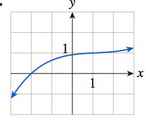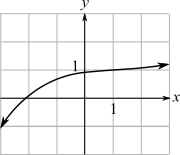Chapter 10.5, Problem 28EFinite Mathematics and Applied Cal...

7th Edition
Stefan Waner + 1 other
ISBN: 9781337274203

Solutions

Chapter
SectionFinite Mathematics and Applied Cal...

7th Edition
Stefan Waner + 1 other
ISBN: 9781337274203
Textbook Problem

In Exercises 27–30, find the approximate coordinates of all points (if any) where the slope of the tangent is (a) 0, (b) 1, and (c) − 1 . [HINT: See Quick Example 3.](a)

To determine

The coordinates on the graph at that point the slope of tangent is 0 from the provided graph is,Explanation

Given information:

The provided graph is:

The slope of the tangent line is given by the rate of change or derivative on the graph of the function f at the particular point x=a.

The tangent line in the graph of function f at the point P(a,f(a)) which is a straight line passing through point P with slope f(a).

Here, the slope of the tangent line is 0, which means the derivative at the particular point, f(a) is 0

(b)

To determine

The coordinates on the graph that has value of the slope of tangent one. The provided graph is,(c)

To determine

The coordinates on the graph that has value of the slope of tangent 1. The provided graph is:Still sussing out bartleby?

Check out a sample textbook solution.

See a sample solution

The Solution to Your Study Problems

Bartleby provides explanations to thousands of textbook problems written by our experts, many with advanced degrees!

Get Started

Use the guidelines of this section to sketch the curve. y=sinx2+cosx

Single Variable Calculus: Early Transcendentals, Volume I

solve the equation by using the quadratic formula. 139. 2x2 + 4x 3 = 0

Applied Calculus for the Managerial, Life, and Social Sciences: A Brief Approach

Does a square have a an incenter? b a circumcenter?

Elementary Geometry For College Students, 7e

Find all degree solutions. 2cos22cos21=0

Trigonometry (MindTap Course List)

For f(x) = 3x + 1 and g(x) = x, ?

Study Guide for Stewart's Single Variable Calculus: Early Transcendentals, 8th